# Integration by parts

(diff) ← Older revision | Latest revision (diff) | Newer revision → (diff)

One of the methods for calculating integrals. It consists in representing an integral of an expression of the formby an integral of. For a definite integral the formula of integration by parts is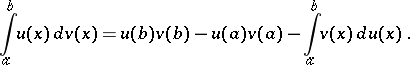(1)

It is applicable under the assumptions that,and their derivatives,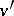are continuous on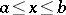.

The analogue of (1) for an indefinite integral is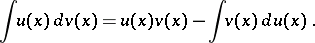(2)

The analogue of (1) for a multiple integral is(3)

Hereis a domain in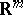with smooth (or at least piecewise-smooth) boundary;; andis the angle between the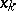-axis and the outward normal to. Formula (3) holds if, e.g.,,and their first-order partial derivatives are continuous on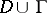. If the integrals in (3) are understood as Lebesgue integrals, then the formula is true ifandbelong to a Sobolev space:,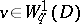for any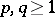with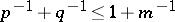.

How to Cite This Entry:
Integration by parts. Encyclopedia of Mathematics. URL: http://encyclopediaofmath.org/index.php?title=Integration_by_parts&oldid=13008
This article was adapted from an original article by V.A. Il'inT.P. Lukashenko (originator), which appeared in Encyclopedia of Mathematics - ISBN 1402006098. See original article